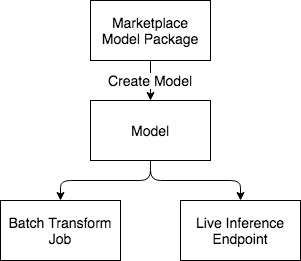# AWS Marketplace Product Usage Demonstration - Model Packages

This notebook’s CI test result for us-west-2 is as follows. CI test results in other regions can be found at the end of the notebook.## Using Model Package ARN with Amazon SageMaker APIs

This sample notebook demonstrates two new functionalities added to Amazon SageMaker: 1. Using a Model Package ARN for inference via Batch Transform jobs / Live Endpoints 2. Using a Marketplace Model Package ARN - we will use Scikit Decision Trees - Pretrained Model

## Overall flow diagram## Compatibility

This notebook is compatible only with Scikit Decision Trees - Pretrained Model sample model that is published to AWS Marketplace

## Set up the environment

[ ]:

import sagemaker as sage
from sagemaker import get_execution_role
from sagemaker.serializers import CSVSerializer

role = get_execution_role()

# S3 prefixes
common_prefix = "DEMO-scikit-byo-iris"
batch_inference_input_prefix = common_prefix + "/batch-inference-input-data"
from sagemaker.predictor import Predictor


### Create the session

The session remembers our connection parameters to Amazon SageMaker. We’ll use it to perform all of our Amazon SageMaker operations.

[ ]:

sagemaker_session = sage.Session()


## Create Model

Now we use the above Model Package to create a model

[ ]:

from src.scikit_product_arns import ScikitArnProvider

modelpackage_arn = ScikitArnProvider.get_model_package_arn(sagemaker_session.boto_region_name)
print("Using model package arn " + modelpackage_arn)

[ ]:

from sagemaker import ModelPackage

model = ModelPackage(
role=role, model_package_arn=modelpackage_arn, sagemaker_session=sagemaker_session
)


## Batch Transform Job

Now let’s use the model built to run a batch inference job and verify it works.

### Batch Transform Input Preparation

The snippet below is removing the “label” column (column indexed at 0) and retaining the rest to be batch transform’s input.

NOTE: This is the same training data, which is a no-no from a statistical/ML science perspective. But the aim of this notebook is to demonstrate how things work end-to-end.

[ ]:

import pandas as pd

## Remove first column that contains the label

TRANSFORM_WORKDIR = "data/transform"

transform_input = (
+ "/batchtransform_test.csv"
)
print("Transform input uploaded to " + transform_input)

[ ]:

import json
import uuid

transformer = model.transformer(1, "ml.m4.xlarge")
transformer.transform(transform_input, content_type="text/csv")
transformer.wait()

print("Batch Transform output saved to " + transformer.output_path)


#### Inspect the Batch Transform Output in S3

[ ]:

from urllib.parse import urlparse

parsed_url = urlparse(transformer.output_path)
bucket_name = parsed_url.netloc
file_key = "{}/{}.out".format(parsed_url.path[1:], "batchtransform_test.csv")

s3_client = sagemaker_session.boto_session.client("s3")

response = s3_client.get_object(Bucket=sagemaker_session.default_bucket(), Key=file_key)
print(response_bytes)


## Live Inference Endpoint

Now we demonstrate the creation of an endpoint for live inference

[ ]:

endpoint_name = "scikit-model"
predictor = model.deploy(1, "ml.m4.xlarge", endpoint_name=endpoint_name)


### Choose some data and use it for a prediction

In order to do some predictions, we’ll extract some of the data we used for training and do predictions against it. This is, of course, bad statistical practice, but a good way to see how the mechanism works.

[ ]:

TRAINING_WORKDIR = "data/training"

import itertools

a = [50 * i for i in range(3)]
b = [40 + i for i in range(10)]
indices = [i + j for i, j in itertools.product(a, b)]

test_data = shape.iloc[indices[:-1]]
test_X = test_data.iloc[:, 1:]
test_y = test_data.iloc[:, 0]

[ ]:

predictor = Predictor(
endpoint_name=endpoint_name, sagemaker_session=None, serializer=CSVSerializer()
)

[ ]:

print(predictor.predict(test_X.values).decode("utf-8"))


### Cleanup endpoint

[ ]:

model.sagemaker_session.delete_endpoint(endpoint_name)
model.sagemaker_session.delete_endpoint_config(endpoint_name)
model.delete_model()


## Notebook CI Test Results

This notebook was tested in multiple regions. The test results are as follows, except for us-west-2 which is shown at the top of the notebook.